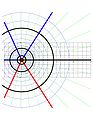# File:Logez02.jpg

Full resolution(612 × 792 pixels, file size: 69 KB, MIME type: image/jpeg)

This file is from a shared repository and may be used by other projects. The description on its file description page there is shown below.

 Description Function$~f=\ln(z)~$ in the complex$~z~$-plane. Abscissa is$~\Re(z)~$, Ordinate is$~\Im(z)~$. lines of constant$~\Re(f)~$ and lines of constant$~\Im(f)~$ are shown. Level$~\Im(f)=-2~$ and level$~\Im(f)=-1~$ are shown with thick red lines. Level$~\Im(f)=1~$ and level$~\Im(f)=2~$ are shown with thick blue lines. Levels$~\Im(f)=0~$ ,$~\Re(f)=-2~$ ,$~\Re(f)=-1~$ ,$~\Re(f)=0~$ ,$~\Re(f)=1~$ ,$~\Re(f)=2~$ , are also shown with thick black lines. Intermediate levels are shown with lines: green is used for$~\Im(f)~=\rm const~$ , red is used for$~\Re(f)~=\rm const < 0~$ , blue is used for$~\Re(f)~=\rm const > 0~$ . The cut at$~z<0~$ is shown with multicolored line. Gridlines are drawn with unity interval and cover the range$~-4\le \Re(z)\le 16~$ ,$~-4\le \Im(z)\le 4~$ . Date 2008 Source Own work Author Domitori Permission (Reusing this file) Free use, distribute, modify. I can even send the source used to plot it.The copyright holder of this file allows anyone to use it for any purpose, provided that the copyright holder is properly attributed. Redistribution, derivative work, commercial use, and all other use is permitted.

## File history

Click on a date/time to view the file as it appeared at that time.

 Date/Time Thumbnail Dimensions User Comment current 04:53, 8 May 2008612×792 (69 KB) Domitori ({{Information |Description= UW PICO(tm) 4.6 File: ogari.txt Funciton ~f=ln(z)~[/itex] in the complex [itex]~z~-plane.
Abscissa is [itex]~\R)

The following 2 pages link to this file: